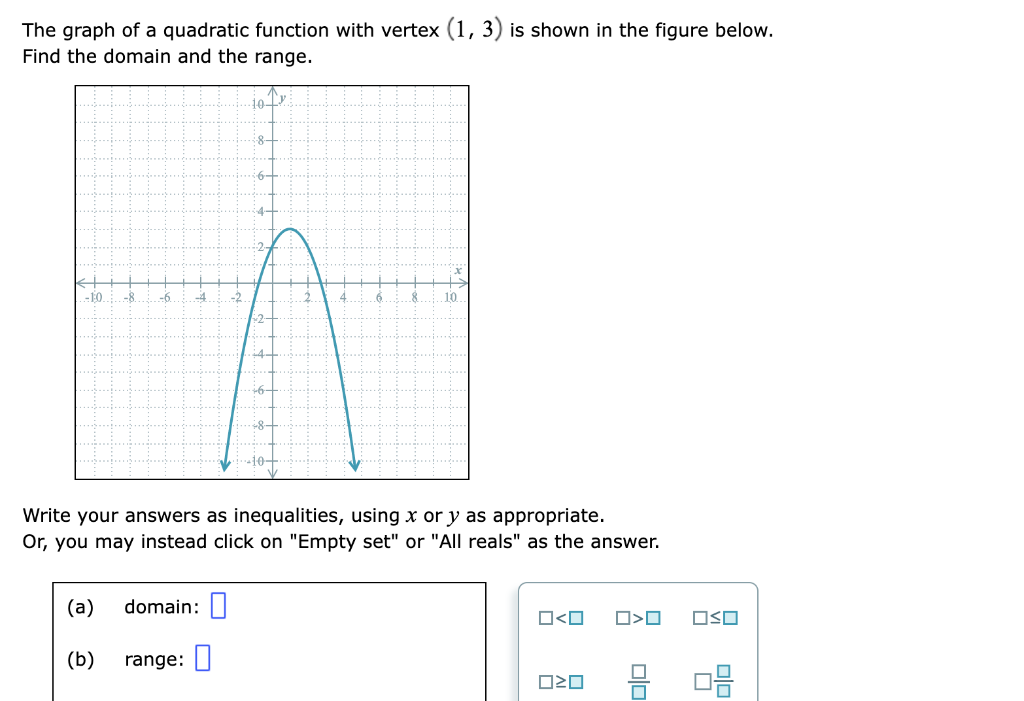# Question Solved1 Answer The graph of a quadratic function with vertex $$(1,3)$$ is shown in the figure below. Find the domain and the range. Write your answers as inequalities, using $$x$$ or $$y$$ as appropriate. Or, you may instead click on "Empty set" or "All reals" as the answer. The graph of a quadratic function with vertex (1,3) is shown in the figure below. Find the domain and the range. Write your answers as inequalities, using x or y as appropriate. Or, you may instead click on "Empty set" or "All reals" as the answer.G8VATG The Asker · Other MathematicsTranscribed Image Text: The graph of a quadratic function with vertex (1,3) is shown in the figure below. Find the domain and the range. Write your answers as inequalities, using x or y as appropriate. Or, you may instead click on "Empty set" or "All reals" as the answer.
More
Transcribed Image Text: The graph of a quadratic function with vertex (1,3) is shown in the figure below. Find the domain and the range. Write your answers as inequalities, using x or y as appropriate. Or, you may instead click on "Empty set" or "All reals" as the answer.